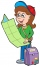# Calculator comparing fractions

The calculator performs basic and advanced operations with fractions, expressions with fractions combined with integers, decimals, and mixed numbers. It also shows detailed step-by-step information about the fraction calculation procedure. Solve problems with two, three, or more fractions and numbers in one expression.

# Calculator comparing fractions

### 1/4 < 2/3

1/4 is less than 2/3

### 0.25 < 0.666666666667

#### Rules for expressions with fractions:

Fractions - use the slash “/” between the numerator and denominator, i.e., for five-hundredths, enter 5/100. If you are using mixed numbers, be sure to leave a single space between the whole and fraction part.
The slash separates the numerator (number above a fraction line) and denominator (number below).

Mixed numerals (mixed fractions or mixed numbers) write as non-zero integer separated by one space and fraction i.e., 1 2/3 (having the same sign). An example of a negative mixed fraction: -5 1/2.
Because slash is both signs for fraction line and division, we recommended use colon (:) as the operator of division fractions i.e., 1/2 : 3.

Decimals (decimal numbers) enter with a decimal point . and they are automatically converted to fractions - i.e. 1.45.

The colon : and slash / is the symbol of division. Can be used to divide mixed numbers 1 2/3 : 4 3/8 or can be used for write complex fractions i.e. 1/2 : 1/3.
An asterisk * or × is the symbol for multiplication.
Plus + is addition, minus sign - is subtraction and ()[] is mathematical parentheses.
The exponentiation/power symbol is ^ - for example: (7/8-4/5)^2 = (7/8-4/5)2

The calculator follows well-known rules for order of operations. The most common mnemonics for remembering this order of operations are:
PEMDAS - Parentheses, Exponents, Multiplication, Division, Addition, Subtraction.
BEDMAS - Brackets, Exponents, Division, Multiplication, Addition, Subtraction
BODMAS - Brackets, Of or Order, Division, Multiplication, Addition, Subtraction.
GEMDAS - Grouping Symbols - brackets (){}, Exponents, Multiplication, Division, Addition, Subtraction.
Be careful, always do multiplication and division before addition and subtraction. Some operators (+ and -) and (* and /) has the same priority and then must evaluate from left to right.

## Fractions in word problems:

• Comparing and sortingArrange in descending order this fractions: 2/7, 7/10 & 1/2
• Equivalent fractionsAre these two fractions equivalent -4/9 and 11/15?
• Ten fractionsWrite ten fractions between 1/3 and 2/3
• CompareCompare fractions (34)/(3) and (12)/(4). Which fraction of the lower?
• FractionsSort fractions z1 = (6)/(11); z2 = (10)/(21); z3 = (19)/(22) by its size. Result write as three serial numbers 1,2,3.
• FractionFind for what x fraction (-4x -6)/(x) equals:
• ArrangeArrange the following in descending order: 0.32, 2on5, 27%, 1 on 3
• 5 books5 books cost \$28.75. Justin wants to buy 8 similar books. He has \$50. Does he have enough?
• DividendsThe three friends divided the win by the invested money. Karlos got three-eighths, John 320 permille, and the rest got Martin. Who got the most and which the least?
• A laundryMr. Green washed 1/4 of his laundry. His son washed 3/7 of it. Who washed most of the laundry? How much of the laundry still needs to be washed?
• Leo hikedLeo hiked 6/7 of a kilometer. Jericho hiked 2/3 kilometer. Who covered a longer distance? How much longer?
• How manyHow many integers are greater than 547/3 and less than 931/4?
• BuingBrother got to buy 240 CZK and could buy for 1/8 what he wanted. Could he pay the rest of the purchase for 200 CZK?